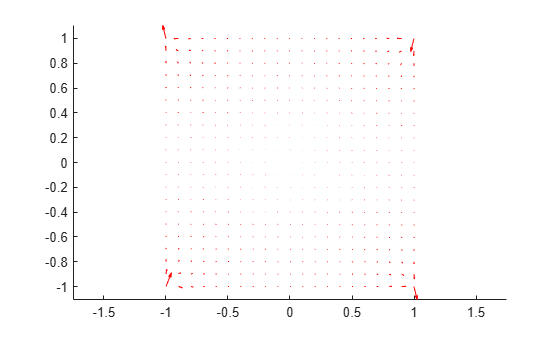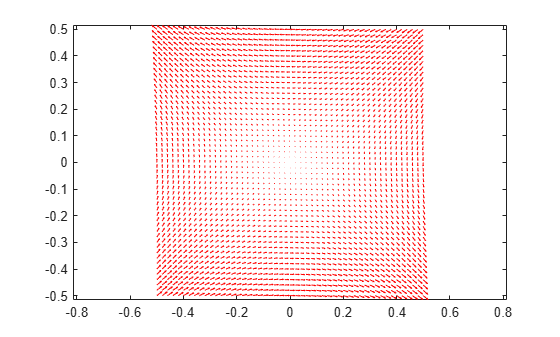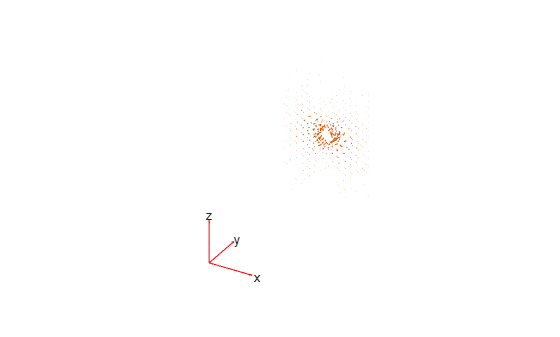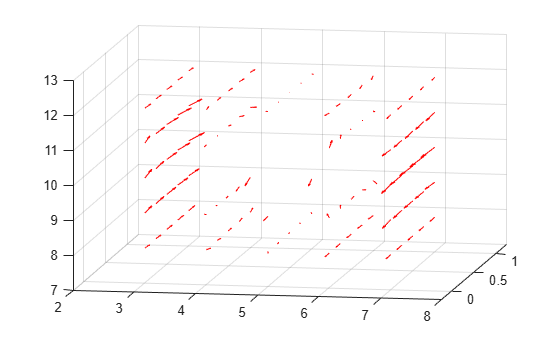interpolateMagneticField

Interpolate magnetic field in magnetostatic result at arbitrary spatial locations

Description

example

Hintrp = interpolateMagneticField(magnetostaticresults,xq,yq) returns the interpolated magnetic field values at the 2-D points specified in xq and yq.

example

Hintrp = interpolateMagneticField(magnetostaticresults,xq,yq,zq) uses 3-D points specified in xq, yq, and zq.

example

Hintrp = interpolateMagneticField(magnetostaticresults,querypoints) returns the interpolated magnetic field values at the points specified in querypoints.

Examples

collapse all

Create an electromagnetic model for magnetostatic analysis.

emagmodel = createpde('electromagnetic','magnetostatic');

Create a square geometry and include it in the model. Plot the geometry with the edge labels.

R1 = [3,4,-1,1,1,-1,1,1,-1,-1]';
g = decsg(R1,'R1',('R1')');
geometryFromEdges(emagmodel,g);
pdegplot(emagmodel,'EdgeLabels','on')
xlim([-1.5 1.5])
axis equalSpecify the vacuum permeability in the SI system of units.

emagmodel.VacuumPermeability = 1.2566370614E-6;

Specify the relative permeability of the material.

electromagneticProperties(emagmodel,'RelativePermeability',5000);

Apply the magnetic potential boundary conditions on the boundaries of the square.

electromagneticBC(emagmodel,'MagneticPotential',0,'Edge',[1 3]);
electromagneticBC(emagmodel,'MagneticPotential',0.01,'Edge',[2 4]);

Specify the current density for the entire geometry.

electromagneticSource(emagmodel,'CurrentDensity',0.5);

Generate the mesh.

generateMesh(emagmodel);

Solve the model and plot the magnetic field.

R = solve(emagmodel);
pdeplot(emagmodel,'FlowData',[R.MagneticField.Hx ...
R.MagneticField.Hy])
axis equalInterpolate the resulting magnetic field to a grid covering the central portion of the geometry, for x and y from -0.5 to 0.5.

v = linspace(-0.5,0.5,51);
[X,Y] = meshgrid(v);

Hintrp = interpolateMagneticField(R,X,Y)
Hintrp =
FEStruct with properties:

Hx: [2601x1 double]
Hy: [2601x1 double]

Reshape Hintrp.Hx and Hintrp.Hy and plot the resulting electric field.

HintrpX = reshape(Hintrp.Hx,size(X));
HintrpY = reshape(Hintrp.Hy,size(Y));

figure
quiver(X,Y,HintrpX,HintrpY,'Color','red')Alternatively, you can specify the grid by using a matrix of query points.

querypoints = [X(:),Y(:)]';
Hintrp = interpolateMagneticField(R,querypoints);

Create an electromagnetic model for magnetostatic analysis.

emagmodel = createpde('electromagnetic','magnetostatic');

Import and plot the geometry representing a plate with a hole.

importGeometry(emagmodel,'PlateHoleSolid.stl');
pdegplot(emagmodel,'FaceLabels','on','FaceAlpha',0.3)Specify the vacuum permeability value in the SI system of units.

emagmodel.VacuumPermeability = 1.2566370614E-6;

Specify the relative permeability of the material.

electromagneticProperties(emagmodel,'RelativePermeability',5000);

Specify the current density for the entire geometry.

electromagneticSource(emagmodel,'CurrentDensity',[0;0;0.5]);

Apply the magnetic potential boundary conditions on the side faces and the face bordering the hole.

electromagneticBC(emagmodel,'MagneticPotential',[0;0;0],'Face',3:6);
electromagneticBC(emagmodel,'MagneticPotential',[0;0;0.01],'Face',7);

Generate the linear mesh.

generateMesh(emagmodel,'GeometricOrder','linear');

Solve the model.

R = solve(emagmodel)
R =
MagnetostaticResults with properties:

MagneticPotential: [1x1 FEStruct]
MagneticField: [1x1 FEStruct]
MagneticFluxDensity: [1x1 FEStruct]
Mesh: [1x1 FEMesh]

Plot the magnetic field density.

pdeplot3D(emagmodel,'FlowData',[R.MagneticField.Hx ...
R.MagneticField.Hy ...
R.MagneticField.Hz])Interpolate the resulting magnetic field to a grid covering the central portion of the geometry, for x, y, and z.

x = linspace(3,7,5);
y = linspace(0,1,5);
z = linspace(8,12,5);
[X,Y,Z] = meshgrid(x,y,z);
Hintrp = interpolateMagneticField(R,X,Y,Z)
Hintrp =
FEStruct with properties:

Hx: [125x1 double]
Hy: [125x1 double]
Hz: [125x1 double]

Reshape Hintrp.Hx, Hintrp.Hy, and Hintrp.Hz.

HintrpX = reshape(Hintrp.Hx,size(X));
HintrpY = reshape(Hintrp.Hy,size(Y));
HintrpZ = reshape(Hintrp.Hz,size(Z));

Plot the resulting magnetic field.

figure
quiver3(X,Y,Z,HintrpX,HintrpY,HintrpZ,'Color','red')
view([30 10])

view([10 15])Input Arguments

collapse all

Solution of a magnetostatic problem, specified as a MagnetostaticResults object. Create magnetostaticresults using the solve function.

Example: magnetostaticresults = solve(emagmodel)

x-coordinate query points, specified as a real array. interpolateMagneticField evaluates the magnetic field at the 2-D coordinate points [xq(i) yq(i)] or at the 3-D coordinate points [xq(i) yq(i) zq(i)] for every i. Because of this, xq, yq, and (if present) zq must have the same number of entries.

interpolateMagneticField converts the query points to column vectors xq(:), yq(:), and (if present) zq(:). It returns magnetic field values as a column vector of the same size. To ensure that the dimensions of the returned solution are consistent with the dimensions of the original query points, use reshape. For example, use HintrpX = reshape(Hintrp.Hx,size(xq)).

Example: xq = [0.5 0.5 0.75 0.75]

Data Types: double

y-coordinate query points, specified as a real array. interpolateMagneticField evaluates the magnetic field at the 2-D coordinate points [xq(i) yq(i)] or at the 3-D coordinate points [xq(i) yq(i) zq(i)] for every i. Because of this, xq, yq, and (if present) zq must have the same number of entries.

interpolateMagneticField converts the query points to column vectors xq(:), yq(:), and (if present) zq(:). It returns magnetic field values as a column vector of the same size. To ensure that the dimensions of the returned solution are consistent with the dimensions of the original query points, use reshape. For example, use HintrpY = reshape(Hintrp.Hy,size(yq)).

Example: yq = [1 2 0 0.5]

Data Types: double

z-coordinate query points, specified as a real array. interpolateMagneticField evaluates the magnetic field at the 3-D coordinate points [xq(i) yq(i) zq(i)]. Therefore, xq, yq, and zq must have the same number of entries.

interpolateMagneticField converts the query points to column vectors xq(:), yq(:), and zq(:). It returns magnetic field values as a column vector of the same size. To ensure that the dimensions of the returned solution are consistent with the dimensions of the original query points, use reshape. For example, use HintrpZ = reshape(Hintrp.Hz,size(zq)).

Example: zq = [1 1 0 1.5]

Data Types: double

Query points, specified as a real matrix with either two rows for 2-D geometry or three rows for 3-D geometry. interpolateMagneticField evaluates magnetic field at the coordinate points querypoints(:,i) for every i, so each column of querypoints contains exactly one 2-D or 3-D query point.

Example: For a 2-D geometry, querypoints = [0.5 0.5 0.75 0.75; 1 2 0 0.5]

Data Types: double

Output Arguments

collapse all

Magnetic field at query points, returned as an FEStruct object with the properties representing the spatial components of the magnetic field at the query points. For query points that are outside the geometry, Hintrp.Hx(i), Hintrp.Hy(i), and Hintrp.Hz(i) are NaN. Properties of an FEStruct object are read-only.